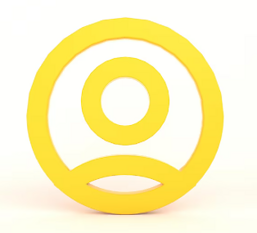(5/5)

### Consider the following program that uses binary search to check whether the input array contains a specific input value.

INSTRUCTIONS TO CANDIDATES

Consider the following program that uses binary search to check whether the input array contains a specific input value. (4*5=20 points)

public class BinarySearch {

public boolean contains(int[] a, int b) {

s1            if (a.length == 0) { //b1(true), b2(false) s2

return false;

}

s3            int low = 0;

s4            int high = a.length - 1;

 s5 s6 while (low <= high) { //b3(true), b4(false) int middle = (low + high) / 2; s7 if (b > a[middle]) { //b5(true), b6(false) s8 low = middle + 1; s9 } else if (b < a[middle]) { //b7(true), b8(false) s10 high = middle - 1; s11 } else { s12 return true; } } s13 return false; } }

1. Draw the control-flow graph of the program
2. Complete the following coverage matrix for both statement (marked on the left side of the code) and branch coverage (marked on the right side of the code) of the following four tests:
3. t1: a={1,2,3}, b=2
4. t2: a={1,2,3}, b=1

iii.    t3: a={1,2,3}, b=3

1. t4: a={1,2,3}, b=4

Statement coerage matrix

Branch coverage matrix

1. Please apply both the total and additional test prioritization (based on statement coverage) on your tests to decide their execution ordering. Please show the priority for each test in each
2. Please apply test-suite reduction (based on the branch coverage) on your tests to remove redundant tests. Please show your encoding of the problem in terms integer linear programming (ILP) constraints and objective formulas, and then manually solve the constraints and generate the reduced test
3. If s10 is changed during software evolution, mark the dangerous edge in the CFG that you drew in (a) and apply regression test selection (RTS) to select the set of affected
(5/5)

## Related Questions

##### Introgramming & Unix Fall 2018, CRN 44882, Oakland University Homework Assignment 6 - Using Arrays and Functions in C

DescriptionIn this final assignment, the students will demonstrate their ability to apply two ma

##### The standard path finding involves finding the (shortest) path from an origin to a destination, typically on a map. This is an

Path finding involves finding a path from A to B. Typically we want the path to have certain properties,such as being the shortest or to avoid going t

##### Develop a program to emulate a purchase transaction at a retail store. This program will have two classes, a LineItem class and a Transaction class. The LineItem class will represent an individual

Develop a program to emulate a purchase transaction at a retail store. Thisprogram will have two classes, a LineItem class and a Transaction class. Th

##### SeaPort Project series For this set of projects for the course, we wish to simulate some of the aspects of a number of Sea Ports. Here are the classes and their instance variables we wish to define:

1 Project 1 Introduction - the SeaPort Project series For this set of projects for the course, we wish to simulate some of the aspects of a number of

##### Project 2 Introduction - the SeaPort Project series For this set of projects for the course, we wish to simulate some of the aspects of a number of Sea Ports. Here are the classes and their instance variables we wish to define:

1 Project 2 Introduction - the SeaPort Project series For this set of projects for the course, we wish to simulate some of the aspects of a number of

### Ask This Assignment To Be Done By Our ExpertsGet A+ Grade Solution Guaranteed##### joyComputer science
(4/5)